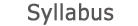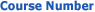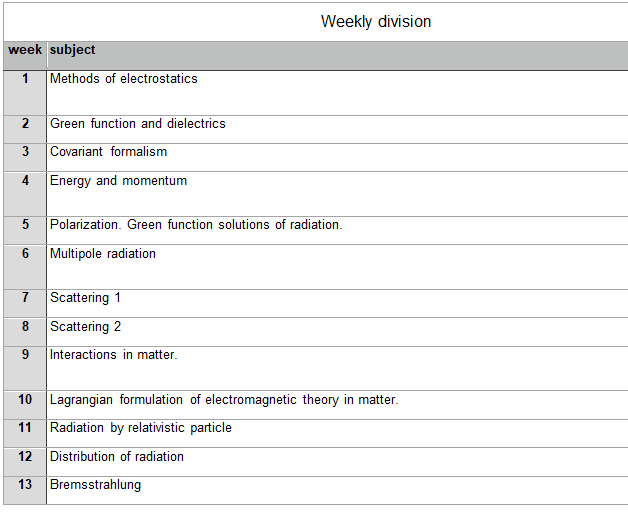0321-4117 Advanced Electromagnetism2019 - 2020View groups
Course descriptionDetailed program

Week 1: Methods of electrostatics

• Overview of methods: images, expansion in 3D and 2D.
• Conformal maps. Green identity.
• Special coordinate systems.

Week 2: Green functions and dielectrics

• Sphere example using images.
• Macroscopic Maxwell equations.
• Dielectric constant, permeability, continuity conditions.

Week 3: Covariant formalism

• Lorentz gauge, Landau gauge, transverse and longitudinal current.
• Relativity theory.
• Tensors, co- and contra-variant, , Maxwell equations in covariant form.

Week 4: Energy and momentum

• Poynting theorem, stress tensor.
• Momentum conservation, stress tensor in mechanics and in Maxwell equations.
• Linear momentum conservation–pressure example, covariant stress tensor definition. Application to plane waves.

Week 5: Polarization; Green functions

• Polarization, Stokes parameters.
• Green function solutions.
• Green function solutions for radiation.

• Dipole radiation; example of charge in circular orbit.

Week 7: Scattering 1

• Main concepts, scattering from a sphere.
• Thompson scattering and Compton effect.
• Scattering from bound electron and from collection of scatterers.

Week 8: Scattering 2

• Perturbation theory in scattering.
• Absorption cross section.
• Optical theorem.

Week 9: Interactions in matter

• Transmission through a slab, relation between forward scattering amplitude and dielectric constant.
• Dynamics of relativistic particles – Lagrangian formulation.
• Spin in magnetic field, spin-orbit coupling, Thomas precession (semi-qualitative treatment).

Week 10: Lagrangian formulation of electromagnetic theory in matter

• Formulation in continuous system – general theory.
• Formulation of EM field in presence of currents.
• Solution of wave equation in covariant form, Green function.

Week 11: Radiation of relativistic particle

• Lienard-Wiechert potentials and fields.
• Non-relativistic limit of Lienard-Wiechert formulas. Larmor formula.
• Total radiation by accelerated particle, relativistic Larmor formula, radiation in linac and cyclotron.